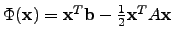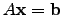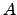• The method of steepest descent'' [251,252] is a iterative optimization method and uses the negative gradient of the function as search direction and combine that with a line search algorithm. However, when the condition number of the system matrix is large, the convergence speed is drastically reduced.
• The conjugate gradient'' (CG) algorithm [219,253] is used to optimize for instance the matrix function, which is equivalent to solving, whereis a symmetric, positive definite system matrix. Other CG variants are theBiconjugate gradient'' method [254,255] and conjugate gradient squared'' method  were introduced to deal with not symmetric or even with non-positive definite matrices.